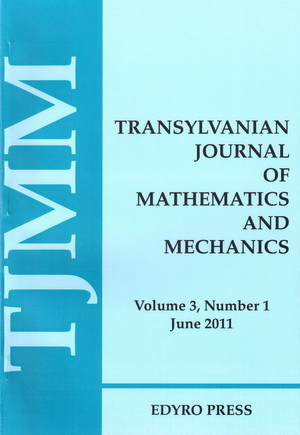ISSN: 2067-239X
ISSN(on-line): 2067-239X

Indexed in:
Mathematical Reviews
Zentralblatt MATH

Front cover# Volume 8 (2016), Number 1

## Hankel Transform in Bicomplex Space and Applications

### Author(s): RITU AGARWAL, MAHESH PURI GOSWAMI and RAVI P. AGARWAL

Abstract: Motivated by the work of Zemanian A.H. in this paper we generalize the complex Hankel transform to bicomplex Hankel transform and derive some of it's basic properties. A table of bicomplex Hankel transform is given for some functions of importance. It has found applications in solving partial differential equation of bicomplex-valued functions, signal processing, optics, electromagnetic field theory and other related problems. The application of Hankel transform has been illustrated by solving bicomplex Cauchy problem.

## A Mathematical Model for the Universe Expansion

### Author(s): MALVINA BAICA and MIRCEA CARDU

Abstract: In this paper we briefly introduce the Universe Expansion Phenomena and we comment about its graphical representation, in the spatial perspective, which appears in the specialized literature. Starting from this spatial representation, we trace the in plane representation of this phenomena. In order to find the mathematical model corresponding to the graphical representation of the Universe expansion we apply the paratrigonometric functions presented in . Finally, we establish the needed mathematical model.

## New Weighted Ostrowski Type Inequalities for Mappings With First Derivatives of Bounded Variation

### Author(s): HÜSEYIN BUDAK and MEHMET ZEKI SARIKAYA

Abstract: In this paper, some new weighted Ostrowski type integral inequalities for mappings whose first derivatives are of bounded variation are obtained and midpoint quadrature formula is provided.

## Weighted Reverse Inequalities of Jensen Type for Functions of Selfadjoint Operators

### Author(s): S.S. DRAGOMIR

Abstract: On making use of the representation in terms of the Riemann-Stieltjes integral of spectral families for selfadjoint operators in Hilbert spaces, we establish here some weighted reverse inequalities of Jensen's type for convex functions of operators. Some applications for simple functions of operators are also provided.

## A Note on New Refinements and Reverses of Young's Inequality

### Author(s): S.S. DRAGOMIR

Abstract: In this note we obtain two new refinements and reverses of Young's inequality.

## On $\left(h-m\right)$-Convexity and Hadamard-Type Inequalities

### Author(s): M. EMIN ÖZDEMIR, AHMET OCAK AKDEMIR and ERHAN SET

Abstract: In this paper, a new class of convex functions as a generalization of convexity which is called $\left(h-m\right)$-convex functions and some properties of this class is given. We also prove some Hadamard's type inequalities.

## Hermite-Hadamard Type Inequalities for the Product Two Differentiable Mappings

### Author(s): A.G. GHAZANFARI and M. SHAFIEI

Abstract: In this paper we extend some estimates of the right hand side of a Hermite-Hadamard type inequality for the product two differentiable functions whose derivatives absolute values are convex. Some natural applications to special weighted means of real numbers are given. Finally, an error estimate for the Simpson's formula is also addressed.

## About Differential Sandwich Theorems Involving a Multiplier Transformation and Ruscheweyh Derivative

### Author(s): ALB LUPAȘ ALINA

Abstract: In this work we study a new operator $I{R}_{\lambda ,l}^{m,n}$ defined as the Hadamard product of the multiplier transformation $I\left(m,\lambda ,l\right)$ and Ruscheweyh derivative ${R}^{n}$, given by $I{R}_{\lambda ,l}^{m,n}:\mathcal{A}\to \mathcal{A}$, $I{R}_{\lambda ,l}^{m,n}f\left(z\right)=\left(I\left(m,\lambda ,l\right)\ast {R}^{n}\right)f\left(z\right)$ and ${\mathcal{A}}_{n}=\left\{f\in ℋ\left(U\right):f\left(z\right)=z+{a}_{n+1}{z}^{n+1}+...,z\in U\right\}$, is the class of normalized analytic functions with ${\mathcal{A}}_{1}=\mathcal{A}$. The purpose of this paper is to derive certain subordination and superordination results involving the operator $I{R}_{\lambda ,l}^{m,n}$ and we establish differential sandwich-type theorems.

## Statistical Analysis of Consumer Price Indices

### Author(s): ANAMARIA POPESCU

Abstract: This paper attempts to characterize through statistical indicators-quantitative measures-chronological series that we have available. The purpose of this paper is to present the calculation of statistical indicators that are used frequently for statistical characterization and adjustment of statistical series time series by identifying the most appropriate statistical model then evolves over time this causes. We can thus analyze central tendency, and data variability, form and concentration distributions package data using analytical tools in Microsoft Excel that enables automatic calculation of descriptive statistics using Data Analysis option from the Tools menu. The analysis of consumer price indices in the period 1990 - 2014 and information website provided by the National Statistics Institute (INS) seems to be a certain tendency towards eccentricity and asymmetry of financial data series.

## Determining Thermoelastic Strains and Stresses Created by the Temperature Field and Constrictions Exerted on the Contours of the Reconditioned Cylindrical Piece

### Author(s): DUMITRU ȘEREMET

Abstract: The main purpose of this article is to determine the distribution of displacements and thermal stresses in composite coatings applied as a compensating layer on reconditioned car parts by means of the squeeze technique. To achieve the purpose means to meet some objectives like: to obtain axial symmetrical displacements and stress fields that are distributed on the thickness of the composite material layer; to validate the received results by solving the boundary value problem by means of the direct integration method and the Green’s function method. As a result of mathematical modeling and theoretical analysis we propose a method to calculate displacements and stresses in different layers of the composite coating, taking into account their constraints too. Analytical expressions and graphs were built for both Green’s functions and heat fields and thermoelastic displacements and stresses for axial symmetrical boundary problems of heat conduction, elastic and thermoelastic theories. The graphic representation has been made using the Maple 15 software. The obtained data can be used for engineering design calculations and choosing appropriate interference fits renovated with composite materials.